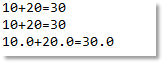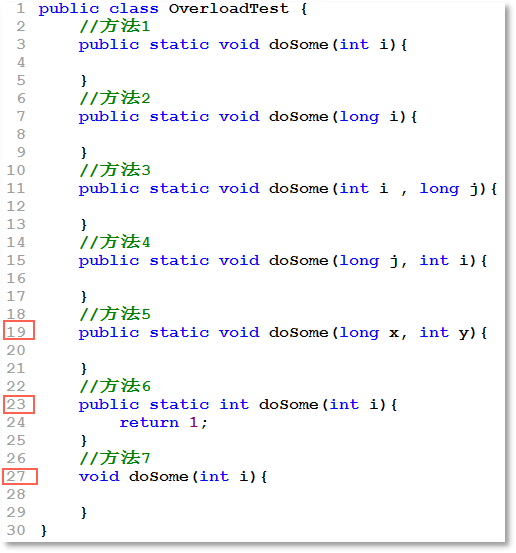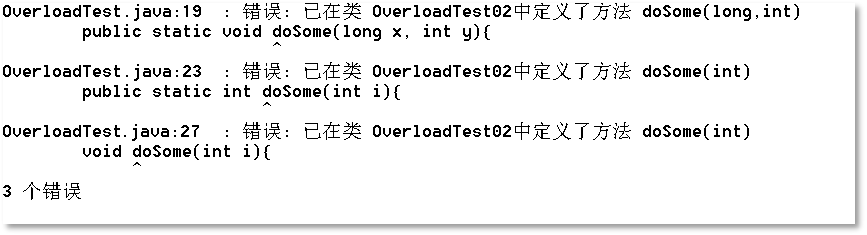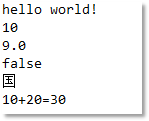# java方法重载/overload

``````public static void main(String[] args) {
int x1 = 10;
int x2 = 20;
int retValue1 = sumInt(x1 , x2);
System.out.println(x1 + "+" + x2 + "=" + retValue1);
long y1 = 10L;
long y2 = 20L;
long retValue2 = sumLong(y1 , y2);
System.out.println(y1 + "+" + y2 + "=" + retValue2);
double z1 = 10.0;
double z2 = 20.0;
double retValue3 = sumDouble(z1, z2);
System.out.println(z1 + "+" + z2 + "=" + retValue3);
}
public static int sumInt(int a , int b){
return a + b;
}
public static long sumLong(long a , long b){
return a + b;
}
public static double sumDouble(double a , double b){
return a + b;
}````````````public static void main(String[] args) {
int x1 = 10;
int x2 = 20;
int retValue1 = sum(x1 , x2);
System.out.println(x1 + "+" + x2 + "=" + retValue1);
long y1 = 10L;
long y2 = 20L;
long retValue2 = sum(y1 , y2);
System.out.println(y1 + "+" + y2 + "=" + retValue2);
double z1 = 10.0;
double z2 = 20.0;
double retValue3 = sum(z1, z2);
System.out.println(z1 + "+" + z2 + "=" + retValue3);
}
public static int sum(int a , int b){
return a + b;
}
public static long sum(long a , long b){
return a + b;
}
public static double sum(double a , double b){
return a + b;
}``````• 在同一个类当中。
• 方法名相同。
• 参数列表不同：个数不同算不同，顺序不同算不同，类型不同也算不同。``````public class U {
public static void p(){
System.out.println();
}
public static void p(int data){
System.out.println(data);
}
public static void p(long data){
System.out.println(data);
}
public static void p(float data){
System.out.println(data);
}
public static void p(double data){
System.out.println(data);
}
public static void p(boolean data){
System.out.println(data);
}
public static void p(char data){
System.out.println(data);
}
public static void p(String data){
System.out.println(data);
}
}``````

``````public class UTest {
public static void main(String[] args) {
U.p("hello world!");
U.p(10);
U.p(9.0);
U.p(false);
U.p('国');
int a = 10;
int b = 20;
int c = a + b;
U.p(a + "+" + b + "=" + c);
}
}``````